Next: Heat Engines Up: Classical Thermodynamics Previous: Van der Waals Gas

# Joule-Thompson Throttling

The difficulty associated with the presence of containing walls can be overcome by replacing the single-event free-expansion process just described (where this one event must supply the energy necessary to change the container temperature) with a continuous-flow process (where the temperature of the walls can adjust itself initially, and remains unchanged after a steady-state has been achieved). We shall now discuss a specific example of such a process that was first suggested by Joule and Thompson.

Consider a pipe with thermally insulated walls. A porous plug in the pipe provides a constriction in the flow. A continuous stream of gas flows through the pipe. The constriction in the flow, due to the presence of the plug, results in a constant pressure difference being maintained across the plug. Let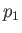andbe the pressure and temperature of the gas upstream of the plug, respectively, and let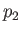and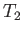be the downstream pressure and temperature, respectively. We expect the upstream pressure to exceed the downstream pressure (i.e.,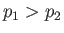), otherwise gas would not be forced through the plug (in the upstream to downstream direction). But, how is the downstream temperature,, related to the upstream temperature,?

Consider a mass of gas,, that flows from the upstream to the downstream side of the plug. In this so-called throttling'' process, the change in internal energy of the mass is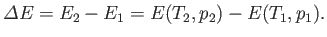(6.167)

However, the mass also does work. Let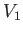and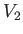be the upstream and downstream volume of the mass, respectively. The mass does the workin displacing gas on the downstream side of the plug, whereas the gas on the upstream side of the plug does the workon the mass. Thus, the net work done by the mass is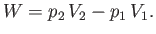(6.168)

No heat is absorbed by the mass of gas in the throttling process that we have just described. This is not just because the walls of the pipe are thermally insulated, so that no heat enters from the outside. More importantly, after a steady-state has been achieved, there is no temperature difference between the walls and the adjacent gas, so there is no heat flow from the walls to the gas. It follows that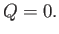(6.169)

Application of the first law of thermodynamics to the mass of gas yields(6.170)

or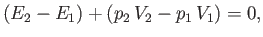(6.171)

where use has been made of Equations (6.167) and (6.168). Now, the enthalpy of the gas mass is defined(6.172)

(See Section 6.10.) Hence, we deduce that(6.173)

or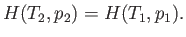(6.174)

In other words, the gas passes through the porous plug in such a manner that its enthalpy remains constant.

Suppose that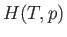is a known function ofand. In this case, givenand, as well as the downstream pressure,, Equation (6.174) allows the downstream temperature,, to be determined. In the case of an ideal gas,(6.175)

so that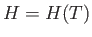is a function of the temperature only. Thus, Equation (6.174) reduces to(6.176)

which implies that. Thus, the temperature of an ideal gas does not change in a throttling process.

For the case of a real gas, the parameter that controls whether the gas is heated or cooled in a throttling process is(6.177)

This parameter is known as the Joule-Thompson coefficient. Given thatdecreases in a throttling process, a positive Joule-Thompson coefficient implies that the temperature also decreases, and vice versa. Let us derive a convenient expression for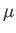in term of readily measured experimental parameters. Starting from the fundamental thermodynamic relation [see Equation (6.5)]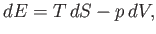(6.178)

we find that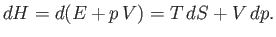(6.179)

For the case of throttling,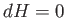. Thus, we can write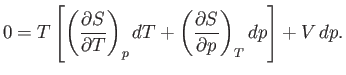(6.180)

With the aid of Equations (6.119) and (6.121), this becomes(6.181)

Hence,(6.182)

where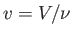is the molar volume.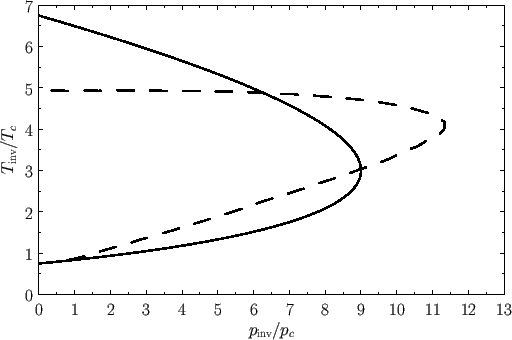Consider a van der Waals gas. The van der Waals equation of state, (6.156), can be written in the reduced form(6.183)

where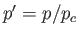,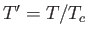, and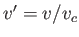. [See Equations (6.158)-(6.160) and Exercise 13.] We can also write(6.184)

where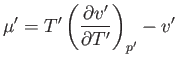(6.185)

Differentiation of Equation (6.183) yields(6.186)

Hence,(6.187)

Thus, we deduce that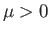, which is consistent with cooling of the gas in a throttling process, provided that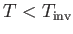, where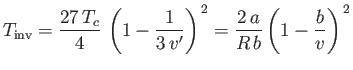(6.188)

is known as the inversion temperature. Of course, if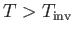then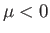, and the gas is heated by a throttling process. Let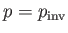when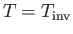. Equations (6.183) and (6.188) give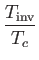(6.189)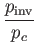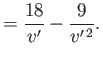(6.190)

These equations define a curve in the-plane, known as the inversion curve, that is shown in Figure 6.2. (Also shown, for comparison, is the experimental inversion curve of nitrogen.) To the left of the curve,, and the gas is cooled by a throttling process. Conversely, to the right of the curve,, and the gas is heated by a throttling process. The maximum possible inversion temperature is. Thus, using the data in Table 6.2, we would predict that the maximum inversion temperatures of helium, hydrogen, nitrogen, and oxygen are 35K, 224K, 852K, and 1044K, respectively. In fact, the experimentally determined maximum inversion temperatures are 34K, 202K, 625K, and 760K, respectively. Thus, our estimate is fairly accurate in the case of helium, but becomes increasingly less accurate as the atomic weight of the gas increases. This is merely a deficiency in the van der Waals model.

The so-called Joule-Thompson throttling process, just described, can be used to cool nitrogen and oxygen to very low temperatures, starting from room temperature, because the maximum inversion temperatures of these two gases both exceed room temperature. On the other hand, an attempt to cool helium gas starting from room temperature would result in an increase, rather than a decrease, in the gas temperature (because room temperature exceeds the maximum inversion temperature). Thus, in order to use the Joule-Thompson process to cool helium gas to very low temperatures, it is first necessary to pre-cool the gas to a temperature that is less than 34K. This can be achieved using liquid hydrogen.Next: Heat Engines Up: Classical Thermodynamics Previous: Van der Waals Gas
Richard Fitzpatrick 2016-01-25